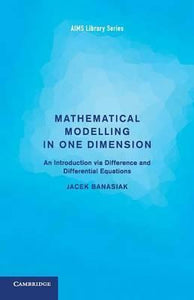# Mathematical Modelling in One Dimension: An Introduction via Difference and Differential Equations by Banasiak, Jacek

• R 220.00
Unit price per
Tax included.

ISBN: 9781107654686

Cambridge University Press | 20 October 2015

Paperback | 122 pages

Mathematical Modelling in One Dimension demonstrates the universality of mathematical techniques through a wide variety of applications. Learn how the same mathematical idea governs loan repayments, drug accumulation in tissues or growth of a population, or how the same argument can be used to find the trajectory of a dog pursuing a hare, the trajectory of a self-guided missile or the shape of a satellite dish. The author places equal importance on difference and differential equations, showing how they complement and intertwine in describing natural phenomena.